Next: Total and effective formation Up: The standard Vesman model Previous: Wave functions

### Decay of the molecular complex

Once the [(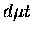)dee] complex is formed, it can either stabilize itself, which leads to fusion, or go through a back decay  into the entrance channel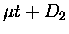: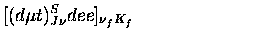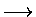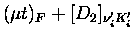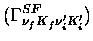(45)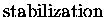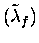(46)

The back decay width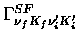is determined by the same matrix element as the formation, though it should be noted that, in general,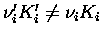(see footnote).

The rate for stabilization, often called the effective fusion rate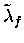, includes contributions from: (1) fusion from the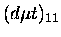state, (2) radiative decay of theinto a lower state, (3) Auger deexcitation of, and (4) collisional deexciation of the complex due to interaction with the surrounding environment. Because of the centrifugal barrier, fusion from J=1 states is relatively slow (< 108 s-1; see Ref.  for a recent summary), and Lane estimates the radiative decay ofto be even slower (s-1 ). Henceis dominated by the Auger deexcitation rate, at least at low densities. This rate has been calculated by several authors with an increasing degrees of sophistication, most recently by Armour et al. , who took into account the molecular nature of the host complex, and estimated the rates for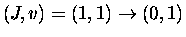to be in the range (6.9-10.3)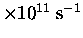, depending on the model used.

The collisional rotational de-exciation of the complex was first calculated by Ostrovskii and Ustimov , and later by Padial, Cohen and Walker . The latter, who claim better accuracy but still neglect the ro-vibrational transitions in the target (i.e., the surrounding) molecule, found the rates substantially smaller than the former (by a factor of two to ten depending on the transition).

To our knowledge, there is no accurate calculation of collisional vibrational quenching, except a rough estimate by Lane of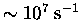at room temperature [151,155], which is much slower than other processes. However, he claims this rate increases drastically with target temperature (for equilibrated targets) and increasing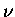, rising to the order of 1010 s-1at 2000 K, therefore it may compete with Auger decay for the molecular formation at epithermal energies with high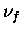(see footnote).

In Faifman's calculations used in our analysis [71,72], the rotational relaxation rate of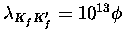s-1, with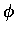being the target density in units of LHD (liquid hydrogen density), and the effective fusion rate of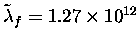s-1 , were used. The latter is the sum of the dipole E1 Auger transition from the (J,v)=(1,1) state to (0,1), (2,0), or (0,0) with each rate being 11.4, 1.3 and 0.02 (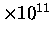s-1), respectively.Next: Total and effective formation Up: The standard Vesman model Previous: Wave functions